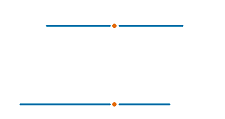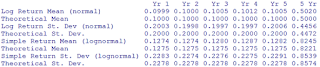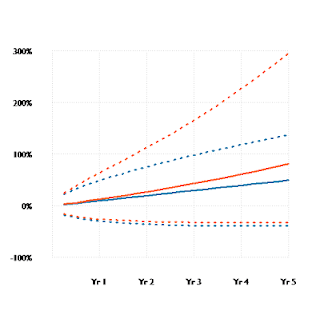## Wednesday, May 22, 2013

### Simulating ROI (Return on Investment) - or What’s so Normal About Logs?

Suppose you and I are in a conference room as members of our organization’s investment committee.
The group next on the agenda walks into the room and takes their seats near the end of the table. They begin outlining their case for an investment proposal they are recommending.
As part

Figure A
Investment Proposal ComparisonSharpe Ratio = Return / St. Dev.
of the underlying analysis, the group ran a Monte-Carlo simulation of their investment (Project A) vs. the base case alternative investment (Project B), with the expected returns and standard deviation of both shown as part of Figure A.

Based on this analysis, Project A has a higher expected return and a more favorable Sharpe ratio, and because of this it is the one recommended for approval.
Should we approve this recommendation?

The Econ Approach
Before answering, let us assume that we and others in our firm are what Daniel Khaneman calls "Econs" – people acting in a completely rational manner consistent with economic theory.
This assumption underlies many finance principles, such as modern portfolio theory, functional capital markets, and investment decisions. We know that the “rational man” assumption is not true in real life, but this may or may not matter. Theories by necessity need to simplify reality (otherwise they would be reality!) and will therefore always contain incorrect assumptions or incomplete representations of reality, but this is acceptable so long as the theory provides actionable explanatory power.
So with respect to the question at hand, investment decisions by Econs simply come down to risk vs. return.

So What’s the Catch?
Given the data in Figure A, along with the Econ stipulation that we focus our decision exclusively on risk vs. return, we are clearly led to the answer: “yes, we should approve Project A”.
But if that is all there is, it wouldn’t be much of a blog post, would it? What is the “catch” to this question?
What dangers are lurking that might cause us to make a mistake in our decision?

In “The Many Faces of ROI” we discussed different
Figure B
Arithmetic Mean and Standard Deviationmethodologies that can be used to calculate a rate of return. In similar fashion does it go with averages.

Figure B shows the equations for the arithmetic average and standard deviation. These are by far the most used and most referred to. This is the calculation where if we ask “what is the average of 2+3+4” we come up with the answer of “3”.
One of the characteristics discussed last time was that returns compound through time – we earn interest on our interest. The fact that this occurs can create complexity when it comes to averages.
Because of compounding, rates of return are not symmetric and can vary a great deal from the arithmetic average.
For example, if we have ²100 (for new readers the symbol ²  stands for Treasury Café Monetary Units, or TCMU’s, freely convertible to any other currency at any exchange rate you choose) and lose 50% the first year and gain 50% the second year, we end up with ²75.
Even though the arithmetic average is 0 ([+50% – 50%] / 2) we have lost 25% of our investment value!
This fact
Figure C
Geometric Mean and Standard Deviationis picked up by what is known as the geometric average. Figure C shows the equations for the geometric average and standard deviation. Note that the geometric average is equivalent to the annualized ROI from our last post.

From Asymmetric to Symmetric
A problem with returns being asymmetric is that it makes it a lot more difficult for us to interpret results within the context of probability distributions. As we have seen, +50% is not the same as -50%, since combined they equal only 75%, yet on a graph both are the same distance away from 0.
We do not naturally look at two points an equal distance away on a line and think of them as being different. So how do we distinguish the difference of the impact of the +50 % vs. the -50%?
The answer: by using the natural
Figure D
Line Length Comparisonlogarithms of the returns (we used the formulas for this in “The Many Faces of ROI”).

Converting the rate of return to its natural logarithm places the ROI into a symmetric context.
Figure D shows 2 lines. The top line represents the +50% and -50%. The bottom line shows the returns after the logarithm conversion. Which set of lines more clearly illustrates the asymmetry to you?

Moving To Normal (and Back Again)
Through the natural logarithm conversion we have taken the asymmetrical and made it symmetrical. This conversion has several other advantages.
§  Returns can be analyzed using a normal distribution, which is more familiar to more people and more studied.
§  There is straightforward method to convert from the symmetric normal distribution to the asymmetric lognormal distribution and back again.
§  We can continue to use many of the finance tools (e.g. option pricing, portfolio management, economic cost of capital) which rely on the normal distribution.
Figure ‘s E and F show the equations to translate the statistical parameters from one distribution to the
Figure E
Converting Normal to Lognormalother.

Figure F
Converting Lognormal to NormalWe now turn to the simulation of investment returns.

Step One: Determine the Distribution and its Parameters
For the first step in the Monte Carlo analysis, we need to determine what type of statistical distribution we are going to use and the parameters of that distribution (mean, standard deviation, etc.).
In last section’s discussion, while we are able to transition either way –  1) from normal to lognormal and 2) from lognormal to normal – there can be problems with the transformation under certain circumstances using the 2nd method, and therefore the 1st is more “error proof”.
So for this post’s example we will use a normal distribution with parameter of 10% average annual return and 20% standard deviation.

Step Two: Determine the Units of Time to Simulate
When dealing with rates of return we need to define time units from two perspectives. First, we need to determine the total time period to be covered. Second, we need to decide how that time period will be divided up.
For example, we might decide we want to simulate a 5 year time frame, and to do it in monthly periods, annual periods, or in one fell 5-year swoop.
This decision will depend in part on the underlying factors that led to the desire to simulate in the first place. In “Should You Rebalance Your Investment Portfolio?” we covered 30 years in monthly increments. Thirty years was chosen as a representative time span of a career. The decision to perform the simulation in monthly increments was driven by the need to replicate frequent periodic contributions to a 401k portfolio or a pension plan. In other situations we may have divided the 30 years differently.
For this post’s example we will choose a 5 year period using monthly increments.

Step Three: Set Up the Simulation Environment
Having made our decisions as to distribution and time frames, we now need to implement these decisions within our simulation environment.
The alternatives for this that I have seen most are Excel (with or without the usage of add-in programs such as @Risk) or one of the
Figure G
Monte Carlo Simulation Equationstatistical software packages (such as SAS, SPSS, or R).

Figure G shows the equations by which the simulation will occur based on our choices so far.
One of the objectives we need to think about when establishing our simulation environment is the information we want to capture. While we may have a primary question we wish to answer, such as “what is the range of returns for a 5 year investment?”, we also are likely to have secondary questions we would like to answer as well, such as “what is the range of quarterly paths this 5 year investment can take?”.
In addition, a good analysis seeks to create mechanisms that allow us to check our work in order to gain confidence in what is produced.
Figure H shows the simulation environment in Excel. You can download this spreadsheet or the R commands if you would like.
Figure H
Excel Simulation EnvironmentStep Four: Simulate
The actual simulation is anti-climatic. We hit a button or execute a command and it happens. Depending on the number of simulations being performed, the amount of data being collected during the process, the amount of computing power we have at our disposal, and other factors it may take a while, so we might use this opportunity to get a cup of coffee, return a phone call, or take a nap!

Step Five: Assess and Analyze Your Results
Once the simulation has been completed we begin the task of assessing and analyzing the results.
As a “check your work” activity, I
Figure I
Year by Year Simulation Resultslike to validate that the simulation has worked in the way intended. Figure I shows the simulation results on a year by year basis along with the total 5 year period. For each period we compare the results generated to their theoretical values. The actual values are within 1/10th of a percentage point to these values, which suggests that we have set up the environment correctly.

This phase of activity will be largely dependent on how much information we stored during the simulation runs and driven by the questions we would like answered.
For our example, suppose that this project will be reported to investors annually, and that we are therefore concerned with the scenario where we will report a loss on the investment. Figure J
Figure J
Probability of Reported Lossshows that this is slightly over 30% for the first year, and that this subsequently declines over the remaining 4 years but is still over 10%.

Going back to the original question at hand, in Figure A we were shown two projects with projected rates of return and standard deviation, and the apparent choice was Project A.
Underlying this conclusion was that the mean and standard deviation parameters are from similar statistical distributions. Suppose we learn that Project A was based on the 5-year average annual returns. This indicates that it was a lognormal distribution since compounding occurs within those 5 years.
Suppose we also learn that Project B’s parameters were based on a consultant’s estimate of annual returns using a normal distribution. This indicates that the data as originally shown is an “apples-to-oranges” situation. Looking at our Monte Carlo results or the equations in Figure E, the lognormal equivalent of this project is a return of 12.75% and a standard deviation of 22.78%, for a Sharpe Ratio of 0.56.
Thus, on an “apples to apples” basis, Project B is superior to Project A!

What Can We Do?
The statistical distribution discrepancy can be the result of many factors. It is not necessarily a product of nefarious manipulation by the promoters of Project A (though it could be!).
More often than not the specification of the distribution is not discussed when we are provided with parameters (for example, JP Morgan’s discussion of asset class returns). In these types of cases we are going to have to draw on our knowledge in other domains in order to decide what is appropriate.
Standardize the Process – within our firm we can standardize the approach by clarifying the statistical distribution to use in order to avoid the “apples and oranges” problem.
Keep Your Thinking Hat On – in some cases a standardized approach may not be possible. When presented with ROI data, we will need to bring our awareness of the differences in distributions in order to ensure we have thought through the results and are satisfied with the conclusions generated.
Verify the Models – often we can use an independent resource within the organization to vet the models and their results. During this process any mixing of distribution assumptions would be discovered and corrected.
Beware of Our Behavioral Biases – due to the asymmetry of the lognormal, results using this appear more biased towards the upside than those of the normal. Figure K
Figure K
Simulation ResultsLognormal returns exhibit assymetry whereas normal returns do not
Dashed lines represent upper and lower bounds of the 95% "zone of confidence"
shows the average returns of our simulation on both a normal and a lognormal basis. Notice how the lognormal is more “hockey stickish” than the normal. Some folks, upon seeing this, will react like the cartoon character with \$ signs spinning in their eyeballs.

Determine the Degree of Believability – no one should base a decision solely on the quantitative output of a simulation or any other analysis. The quantitative information can be valuable, but it is not a substitute for decision making judgment on the part of leadership. It is one factor out of many. Sometimes we use the quantitative to provide an “order of magnitude” assessment rather than a precise forecast. Other times we might know that the distributions used are not entirely representative of reality but have no realistic alternative, and therefore accept the simplifying assumptions of the model used.

Key Takeaways
Returns on Investment (ROI) can be portrayed in different ways using different statistical distributions. Understanding how the normal and the lognormal are related and how they are different provides us with the means necessary to make better informed decisions when assessing quantitative data.

You May Also Like

Questions
§  What experience have you had in your organization or in your own analysis with the lognormal and normal distribution?
§  Have you witnessed blatant misuse of these calculations?
§  What topics would you like to see addressed on this blog?